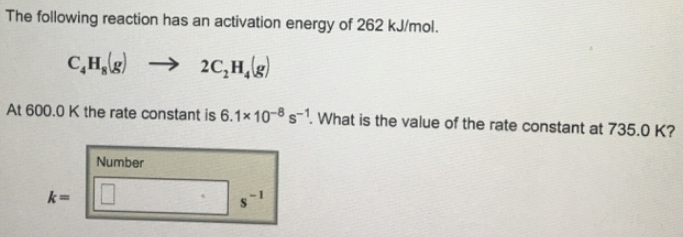# Problem: The following reaction has an activation energy of 262 kJ/mol. C4H8 (g) → 2C2H4 (g) At 600.0 K the rate constant is 6.1 x 10-8 s-1. What is the value of the rate constant at 735.0 K?

###### FREE Expert Solution
93% (142 ratings)###### Problem Details

The following reaction has an activation energy of 262 kJ/mol.

C4H8 (g) → 2C2H4 (g)

At 600.0 K the rate constant is 6.1 x 10-8 s-1. What is the value of the rate constant at 735.0 K?